# # 11 CFL 可达与 IFDS

## # 11.1 可行路径与可实现路径

### # 11.1.1 可行路径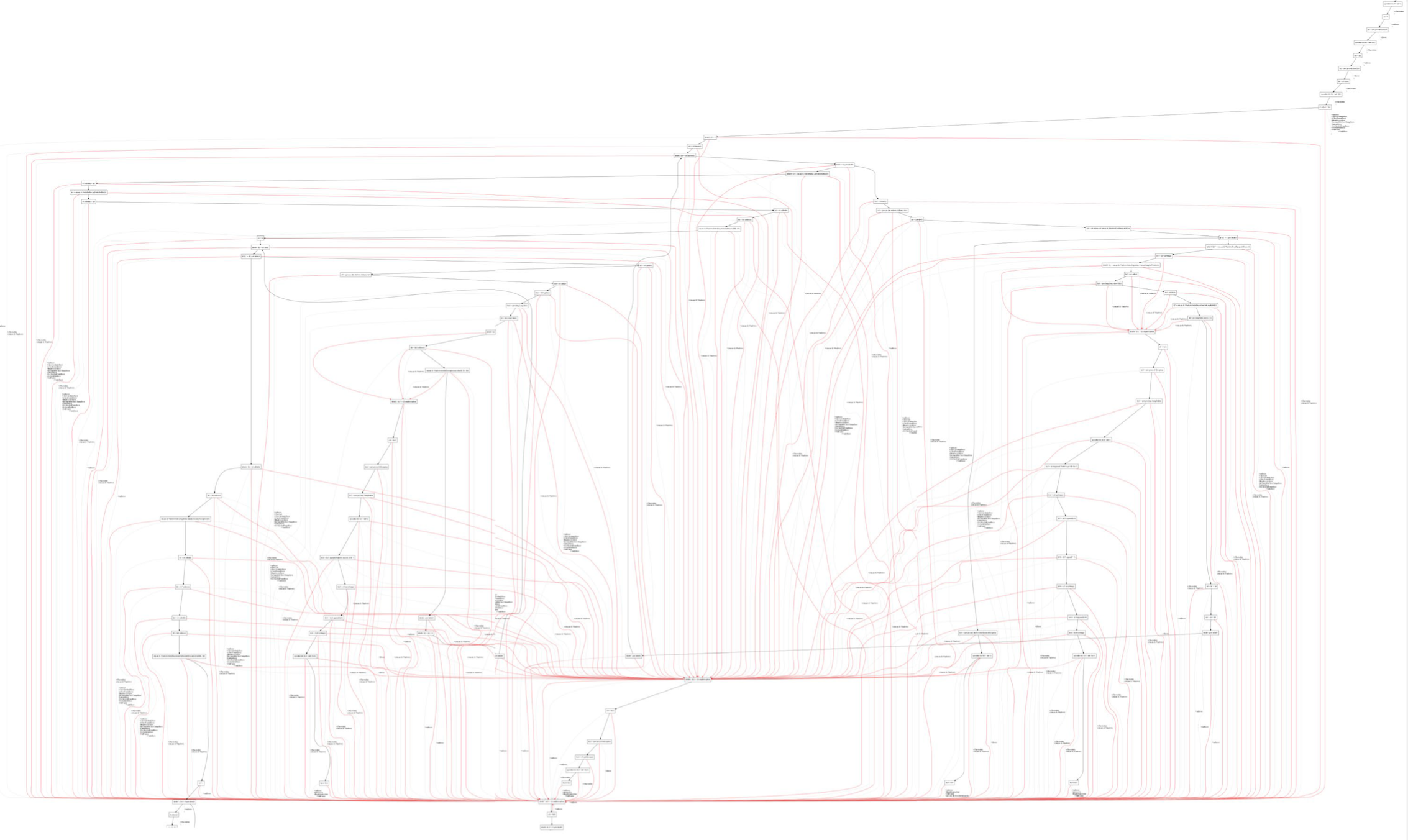void main() {
x = foo(8);
...
y = foo(30);
...
}

int foo(int age) {
if (age >= 0)
r = age;
else
r = -1;
return -1;
}

1
2
3
4
5
6
7
8
9
10
11
12
13
14• -1 是由于下图中标红色的部分产生的，这个假积极是我们无法避免的。

• 因为从静态分析的角度，我们并不知道这个函数的输入是什么，根据安全近似（Safe-Approximation, 见定义 3.1）的原则，我们应该进行过近似（Over-Approximation，见定义 1.3），因此左边和右边的路径都只能保留下来。• 30 是由于下图中标红色的部分产生的，这个假积极是我们可以避免的。

• 因为从 foo(30) 调用点出发的调用控制流，不可能会回到 foo(18) 的返回点，这一点是和程序的输入无关的，是在静态分析的阶段我们就可以确定下来的。

• 言下之意，如果我们能够避免这样的不可行路径，我们至少可以再提高一些静态分析的精度。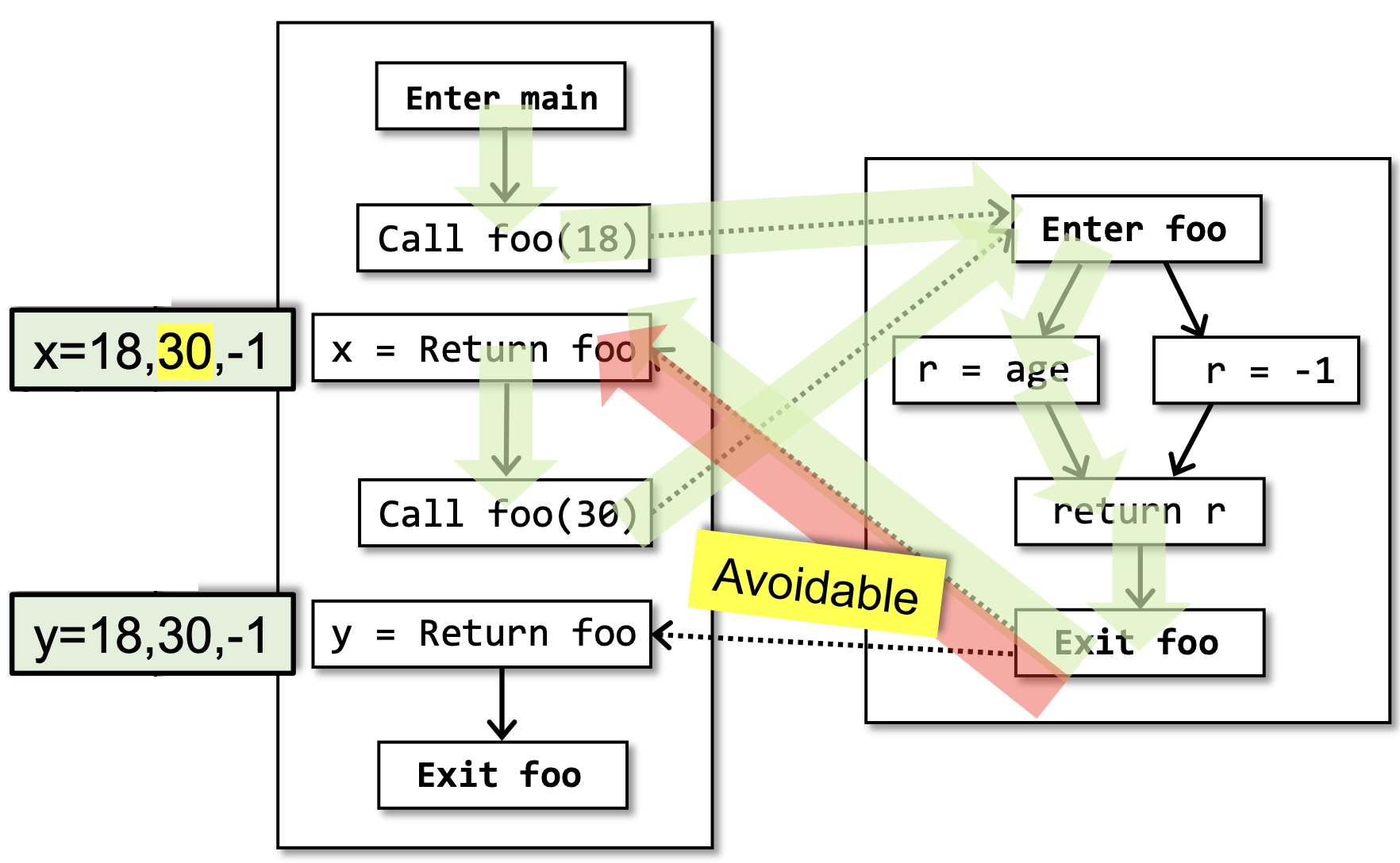### # 11.1.2 可实现路径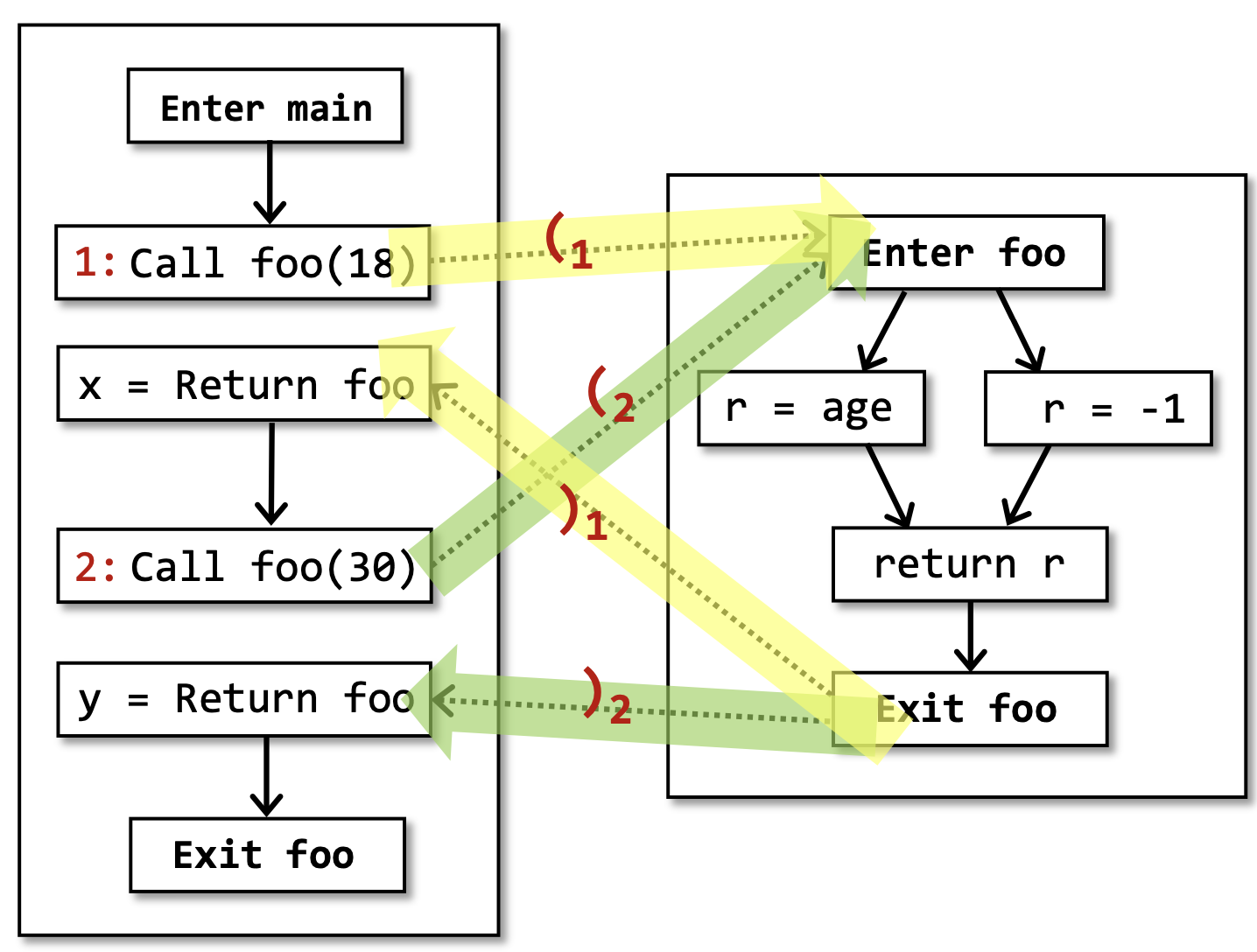## # 11.2 CFL 可达性

### # 11.2.1 上下文无关文法

$L \subseteq \{s| s = a_1a_2...a_n, a_i\in \Sigma, n \in\mathbb{N}\}$

$S \to S'$

\begin{aligned} S &\to S_1\\ &\to S_2\\ &\dots\dots\\ &\to S_n \end{aligned}

$S \to \alpha$

### # 11.2.2 CFL 可达性

• 每个右括号 $)_i$ 都应当有一个左括号 $(_i$ 与之平衡，但反之不亦然；
• 对于每个调用点 $i$ ，将它的调用边标记为 $(_i$ ，返回边标记为 $)_i$
• 将其他所有的边标记为 $e$

\begin{aligned} realizable &\to matched\ realizable\\ &\to (_i\ realizable\\ &\to \varepsilon\\ matched &\to (_i\ matched\ )_i\\ &\to e\\ &\to \varepsilon\\ &\to matched\ matched \end{aligned}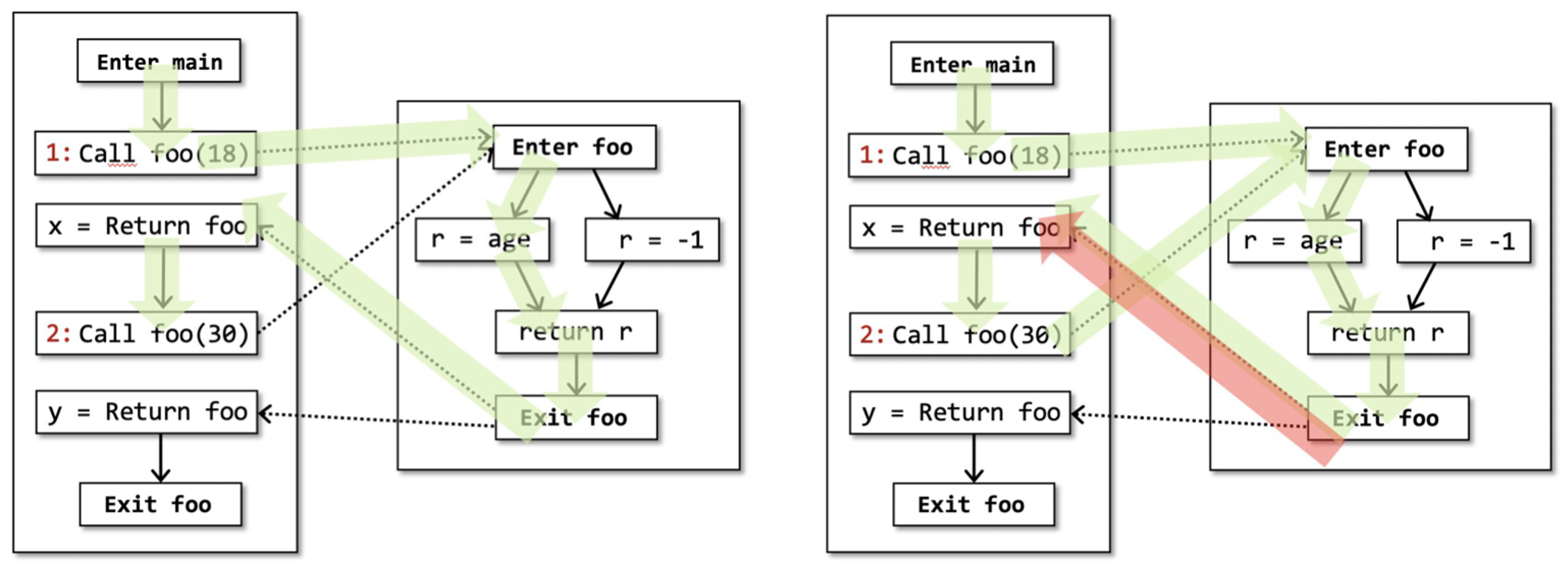## # 11.3 IFDS 概述

### # 11.3.1 概念

IFDS 是一种通过图可达性的方式进行静态程序分析的框架。

“Precise Interprocedural Dataflow Analysis via Graph Reachability” —— Thomas Reps, Susan Horwitz, and Mooly Sagiv, POPL’95

IFDS（Interprocedural Finite Distributive Subset Problem） 指的是一类过程间（Interprocedural）数据流分析的子问题，其流函数具有分配性（Distributive），定义域（Domain，见定义3.5）是有限（Finite）集。

IFDS为数据流分析提供了一种可实现全路汇集（MRP）的解决方案，在定义4.14中，我们定义了全路汇集（MOP）的解决方案，MRP的定义也是类似的。

• 考虑从程序入口到 $s_i$ 处的路径 $P$流函数（Flow Function）$F_P$ ，所有可实现路径的集合记为 $RPaths(ENTRY, s_i)$

• 这里的流函数的作用类似于定义4.14中的状态转移方程，只不过4.14是结点转移，这里说的是边转移（结点转移可以转化成边转移，后续会有例子）。

• 一条路径的流函数，和定理4.10类似，是路径上边的流函数的复合。

• 使用联合（join）或者汇集（meet）操作来求这些值的最小上界或者最大下界。

$MRP[s_i] = \bigvee_{\forall P \in RPaths(ENTRY, s_i)} F_P(OUT[ENTRY])$

$MRP[s_i] = \bigwedge_{\forall P \in RPaths(ENTRY, s_i)} F_P(OUT[ENTRY])$

MRP 比 MOP 更准确（precise），具体地：

• 若进行可能性分析（May Analysis），采用联合操作，则 $MRP[s_i] \preceq MOP[s_i]$

• 若进行必然性分析（Must Analysis），采用汇集操作，则 $MOP[s_i] \preceq MRP[s_i]$

$MRP[s_i] = \bigvee_{\forall P \in RPaths(ENTRY, s_i)} F_P(OUT[ENTRY])\\ \preceq \bigvee_{\forall P \in RPaths(ENTRY, s_i)} F_P(OUT[ENTRY]) \vee \bigvee_{\forall P \in Paths(ENTRY, s_i) - RPaths(ENTRY, s_i)} F_P(OUT[ENTRY])\\ = \bigvee_{\forall P \in Paths(ENTRY, s_i)} F_P(OUT[ENTRY]) = MOP[s_i]$

$MOP[s_i] = \bigwedge_{\forall P \in Paths(ENTRY, s_i)} F_P(OUT[ENTRY])\\ = \bigwedge_{\forall P \in RPaths(ENTRY, s_i)} F_P(OUT[ENTRY]) \wedge \bigwedge_{\forall P \in Paths(ENTRY, s_i) - RPaths(ENTRY, s_i)} F_P(OUT[ENTRY])\\ \preceq \bigwedge_{\forall P \in RPaths(ENTRY, s_i)} F_P(OUT[ENTRY]) = MRP[s_i]$

### # 11.3.2 基本步骤

IFDS分析框架：

• $P$ 建立一个 超图（Supergraph） $G^{*}$ 并且根据 $Q$ 定义 $G^{*}$ 中边的流函数；

• 通过将流函数转化成 代表关系（Representation Relations） 的方式，基于 $G^{*}$$P$ 建立一个 分解超图（Exploded Supergraph） $G^{\sharp}$

• $Q$ 可以被当作图 $G^{\sharp}$ 上的可达性问题来解决（寻找MRP解决方案），具体地，通过在 $G^{\sharp}$ 上运行制表算法来解决。

$n$ 是某个程序点， 数据流因素 $d \in MRP[n_i]$ 当且仅当在 $G^{\sharp}$ 中存在一条从 $(s_{main}, 0)$$(n_i, d)$ 的可实现的路径。

## # 11.4 超图与流函数

### # 11.4.1 超图的构建

• $G^{*}$ 是由一组控制流图（Control Flow Graph，见定义2.4与算法2.2） $G_1, G_2, ...$ 组成的，每个过程 $Procedure_i$ 都有一个对应的控制流图 $G_i$

• 每一个控制流图 $G_p$ 都有自己独特的一个起始结点（Start Node） $s_p$ 和结束结点（Exit Node） $e_p$

• 在每个过程的控制流图 $G_i$ 中，用调用结点（Call Node） $Call_p$ 和 返回点结点（Return-site Node） $Ret_p$ 来表示对 $Procedure_p$ 的过程调用。

• 除了每个过程内部的控制流边以外，对于每个过程调用， $G^{*}$ 还有另外3种边：

• 一条过程内的，从调用者的 $Call_p$$Ret_p$调用返回边（call-to-return-site edge）

• 一条过程间的，从调用者 $Call_p$ 到被调用者的 $s_p$调用起始边（call-to-start-edge）

• 一条过程间的，从被调用者的 $e_p$ 到调用者的 $Ret_p$结束返回边（exit-to-return-edge）

int g;
void main() {
int x;
x = 0;
P(x);
}
void P(int a) {
if (a > 0) {
g = 0;
a = a - g;
P(a);
Print(a, g);
}
}

1
2
3
4
5
6
7
8
9
10
11
12
13
14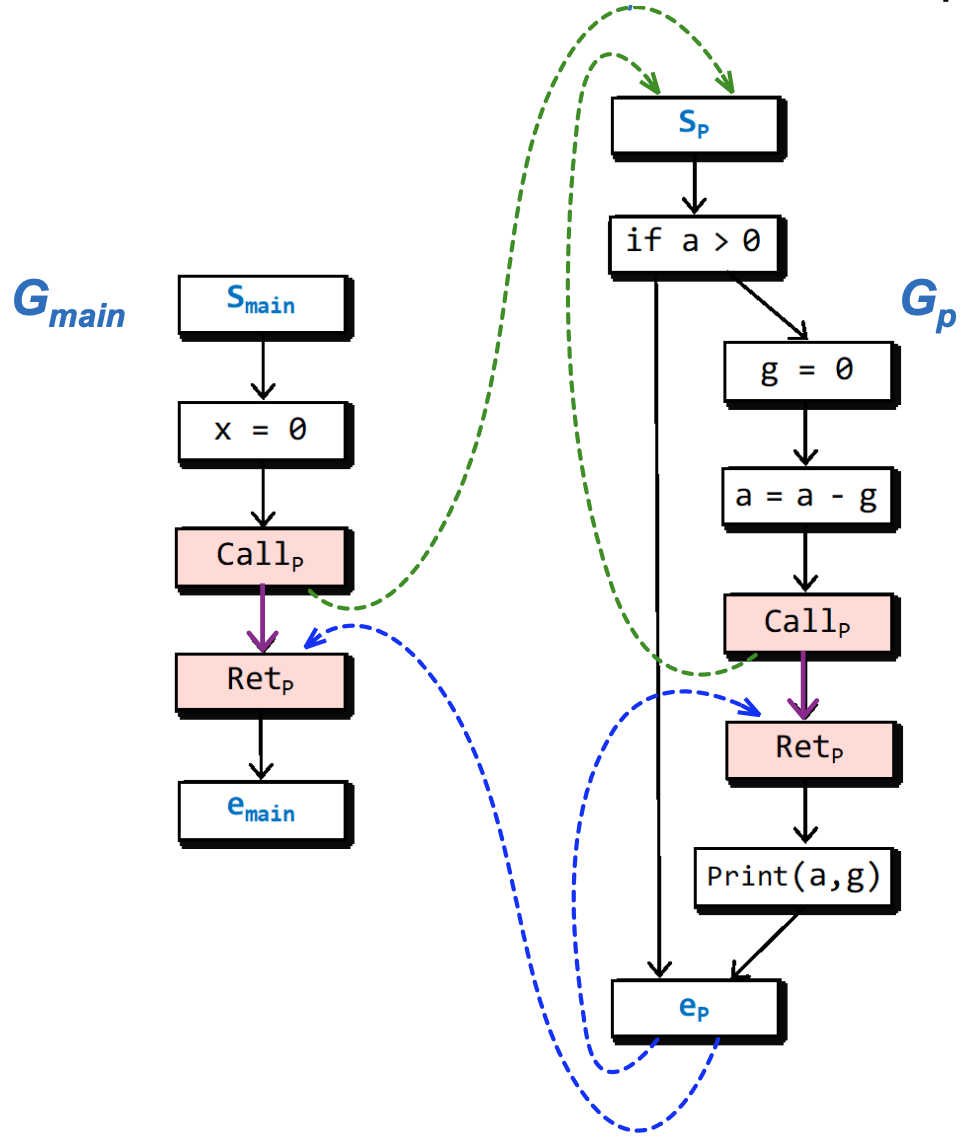• 对于结点，白底蓝字的是起始结点和结束结点，红底黑字的是调用结点和返回结点，白底黑字的是普通结点。

• 对于边，黑色的是普通控制流边，紫色的是调用返回边，绿色的是调用起始边，蓝色的是结束返回边。

### # 11.4.2 流函数的设计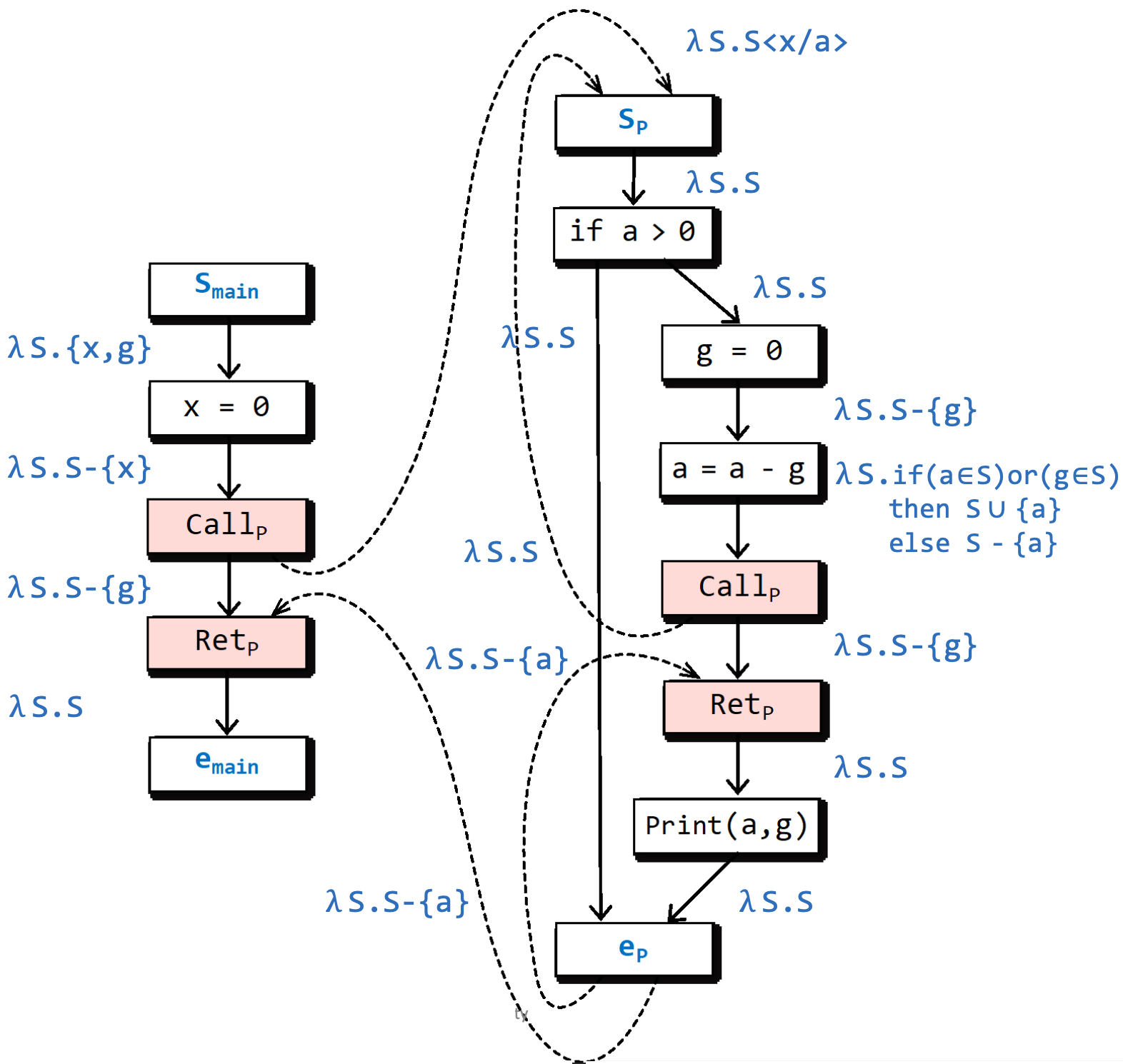• 比如说，我有好多个变量，但是我调用的过程只需要其中一两个，那么剩下的变量就可以不需要进入过程体，直接通过调用返回边传递给后面的结点即可。

• 此外，有些方法的副作用，我们也可以用调用返回边来传递。

• 起点边：定义初始状态；

• 普通边：表达出语句的语义；

• 调用起始边：绑定参数，改名字（rename）；

• 调用返回边：传递本地信息和过程副作用；

• 结束返回边：传递返回值信息和表达本地作用域的消亡。

## # 11.5 分解超图和制表算法

### # 11.5.1 代表关系

$R_f = \{(0, 0)\} \cup \{(0, y)| y \in f(\emptyset)\}\cup\{(x, y)|y \notin f(\emptyset) \wedge y\in f(\{x\})\}$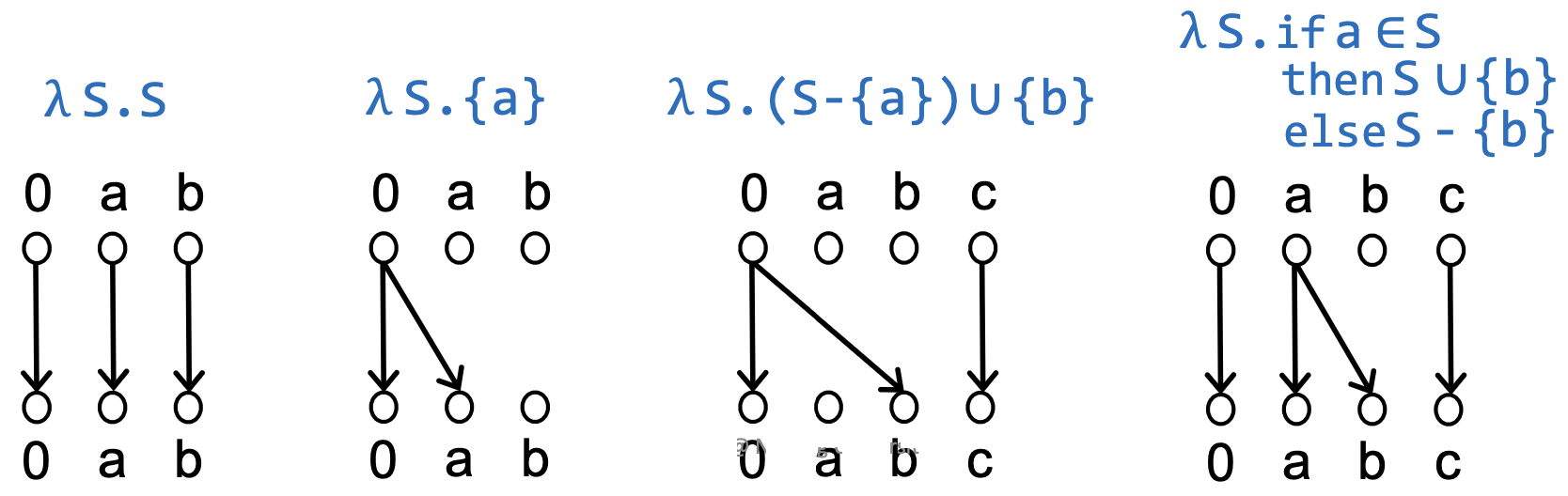### # 11.5.2 构建分解超图

• $V^{\sharp} = \{(n_i, d)|n_i\ is\ a\ program\ point\ and\ d\ is \ a\ dataflow\ factor\}$
• $(n_i, d_1) \to (n_{i + 1}, d_2) \in E^{\sharp} \Leftrightarrow (d_1, d_2) \in R_{f_i}$ ，其中， $f_i$$i$ 处的流函数。### # 11.5.3 关于粘边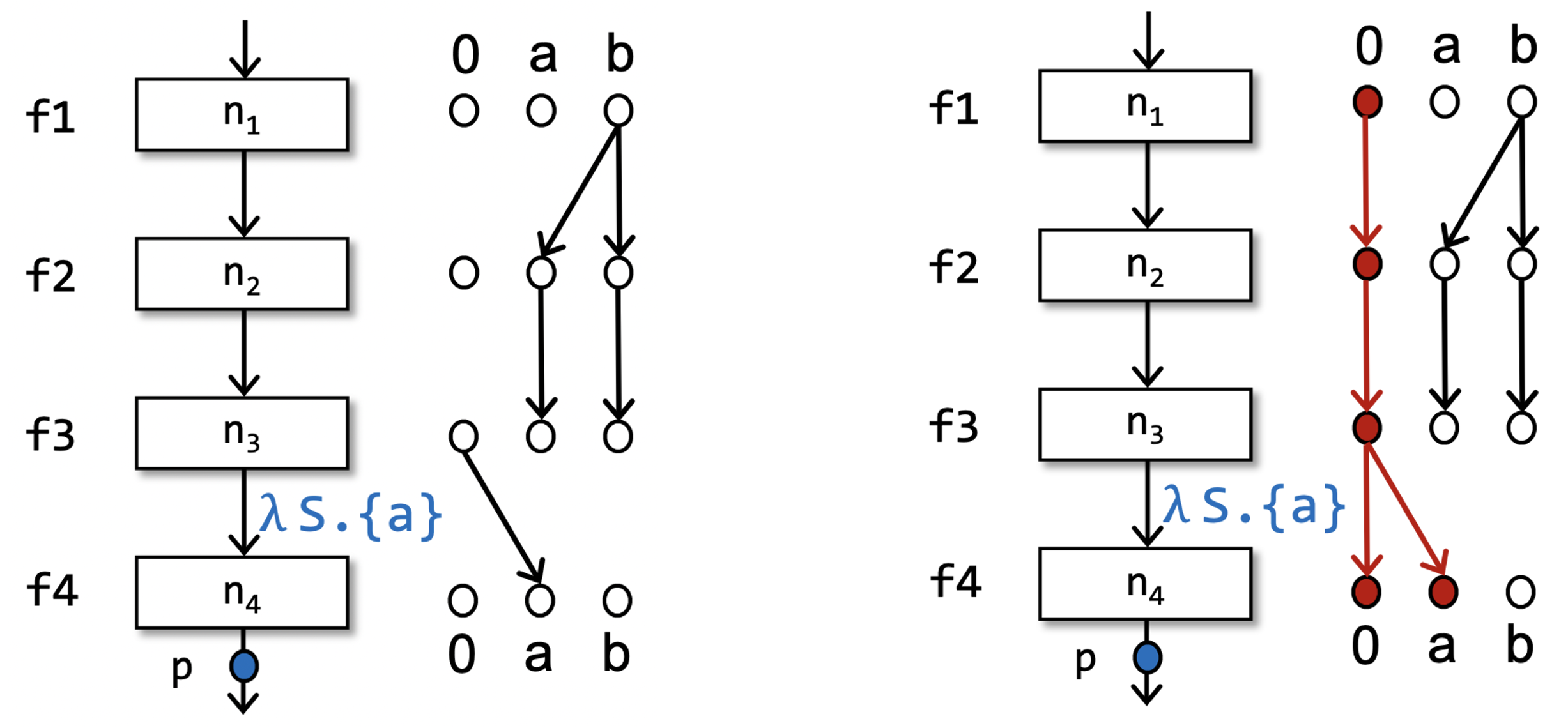### # 11.5.4 制表算法

#### # 直观感知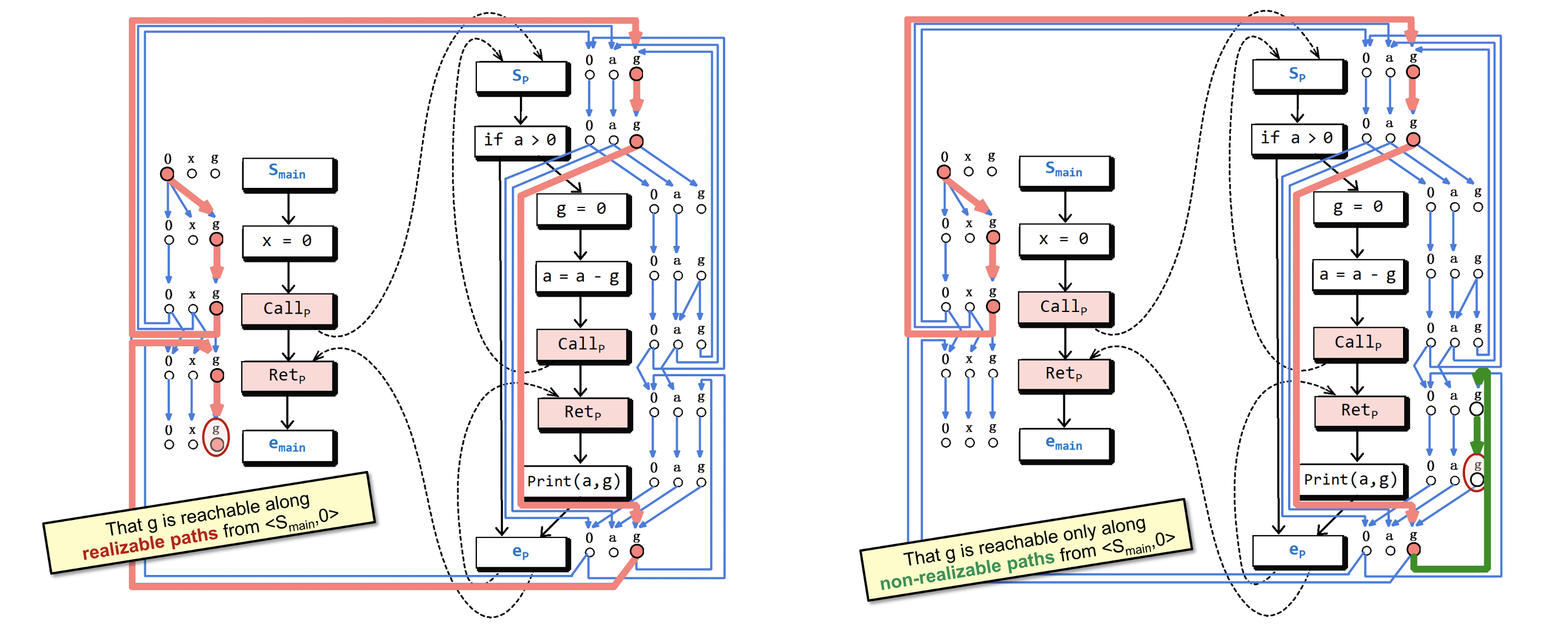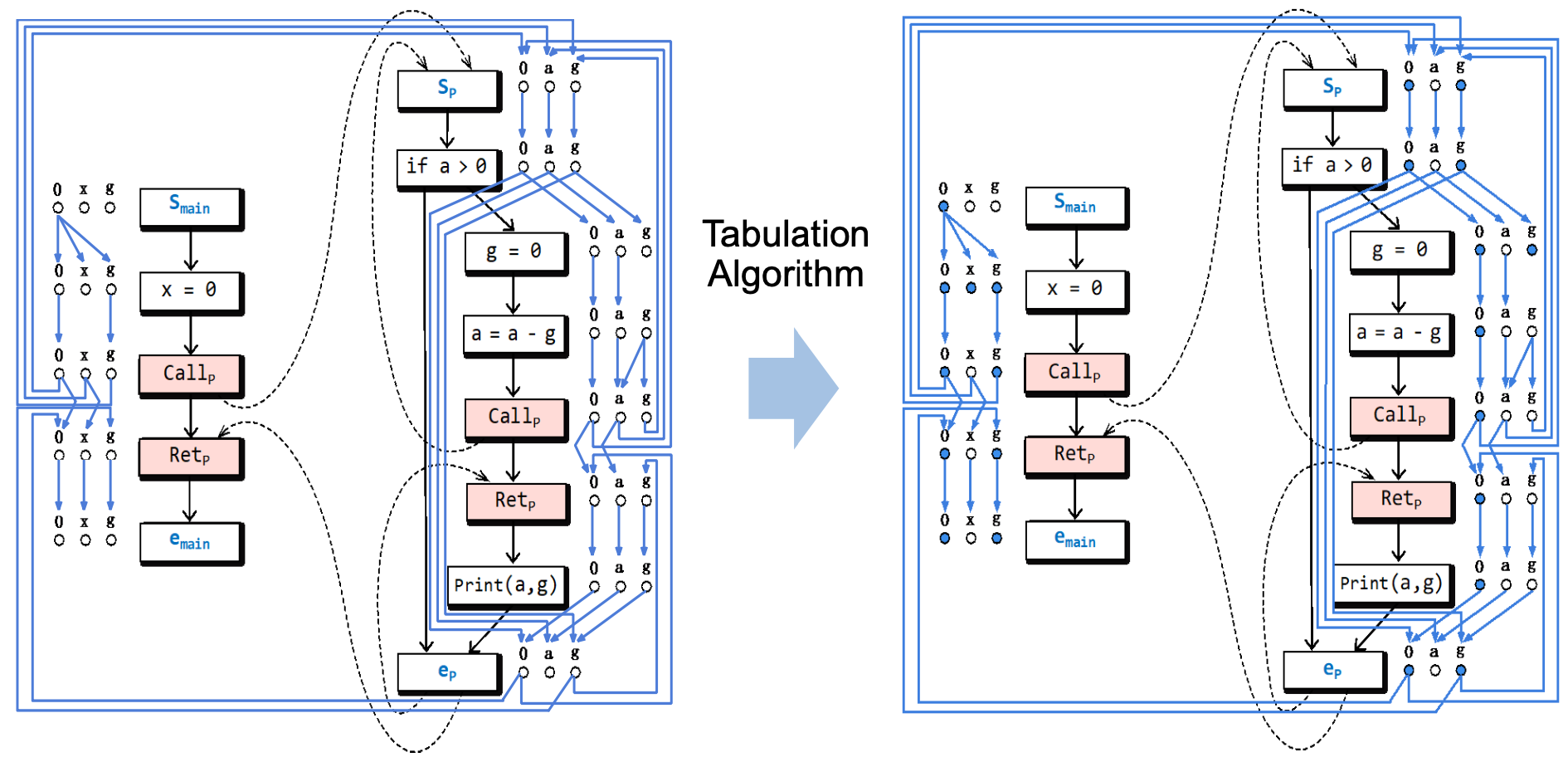#### # 工作原理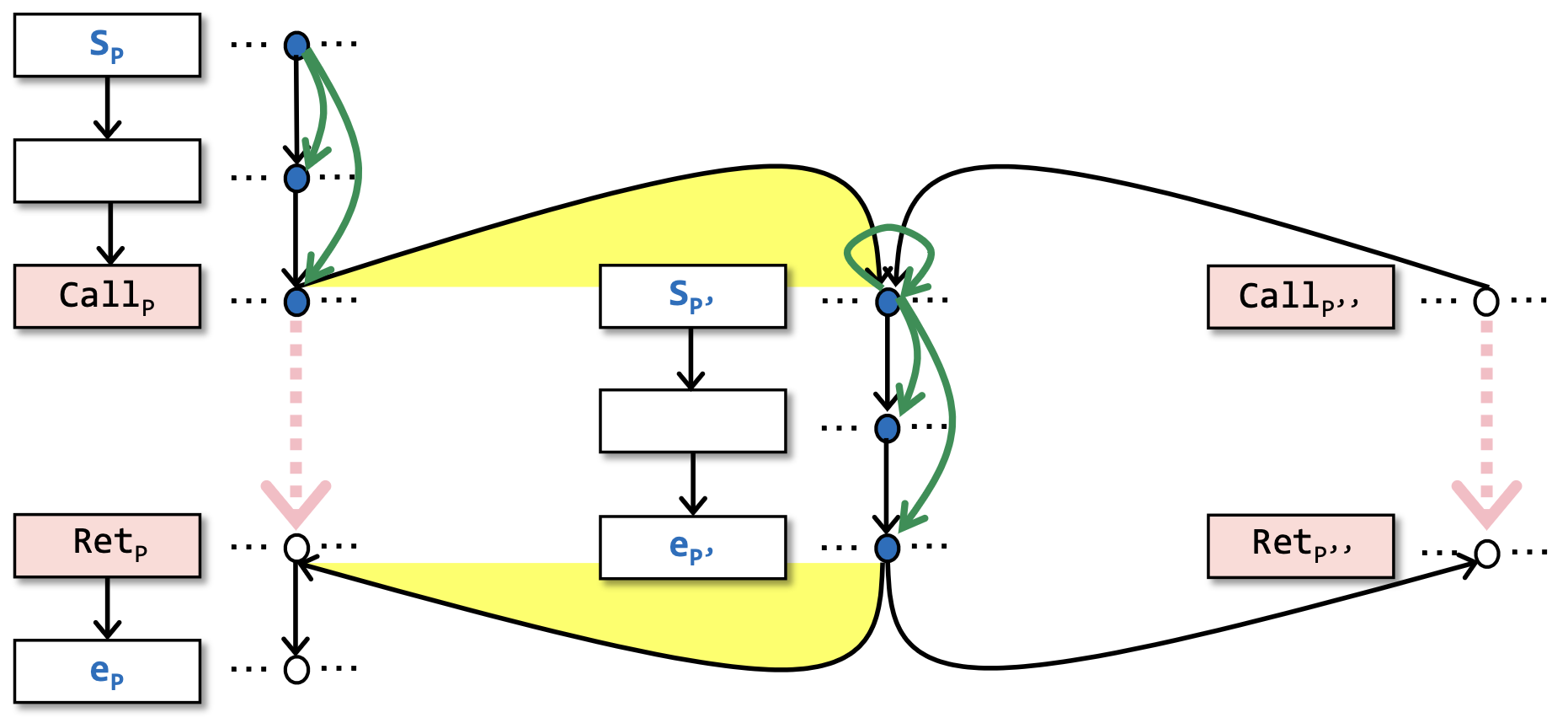1. 在每发现一个方法可达的时候，将可达性分析本地化。

见图上 $S_{p'}$ 出的自引（self-reference）绿边。

• 图上形如 $v_1 \to v_2$ 的绿边表示 $v_2$$v_1$ 可达（注意这里只是一般的可达，并不特指 CFL 可达，CFL 可达是通过后面的 2 和 3 两点来共同保证的）。

• 当一个过程 $S_{p'}$ 被调用的时候，让 $S_{p'}$ 自引表示它一定是被某个过程给调用了，但具体是哪一个过程对可达性来说其实不重要，$p'$ 内部的可达性分析只需要做一遍，当 $p'$ 被调用第二次的时候，发现 $S_{p'}$ 已经自引了，就不需要再分析 $p'$ 内部的可达性了。

• 我们也可以一直维护着一个全局的可达性分析，但这样会有冗余的重复分析，不如对每个可达方法进行局部分析，然后再组合成一个整体的结果来得更高效。

2. 在处理每一个过程的结束结点（Exit Node）的时候，做调用-返回的匹配（Call-to-return Matching）：

见图上黄色部分。

• 先找到所有的调用 $p'$ 的调用点 $(Call_p, Call_{p''})$

• 然后找出它们对应的返回点 $(Ret_{p}, Ret_{p''})$

3. $(Call, d_m)$$(Ret, d_n)$ 的总结边（Summary Edge）表示通过调用方法 $p'$$d_n$ 是从 $d_m$ 可达的。

见图上粉色部分，如果一个方法的开始结点到结束结点可达，会在这个方法所有匹配的调用点和返回点之间添加总结边。

• 在此时，有些方法调用可能还没有被处理，等之后再用到这个方法的时候，有了总结边，我们就知道了这个方法的可达性，从而就不需要再重复地分析一遍这个方法了，能够消除冗余的分析过程。

• 其实，我感觉这里的总结边有点像是一种缓存，用空间换时间，从而提高算法效率。这也是为什么在上面 1 的时候只需要本地化地分析过程内部的可达性就可以了，因为之后可以用总结边来概括这个过程调用的开始结点和结束结点之间的可达性。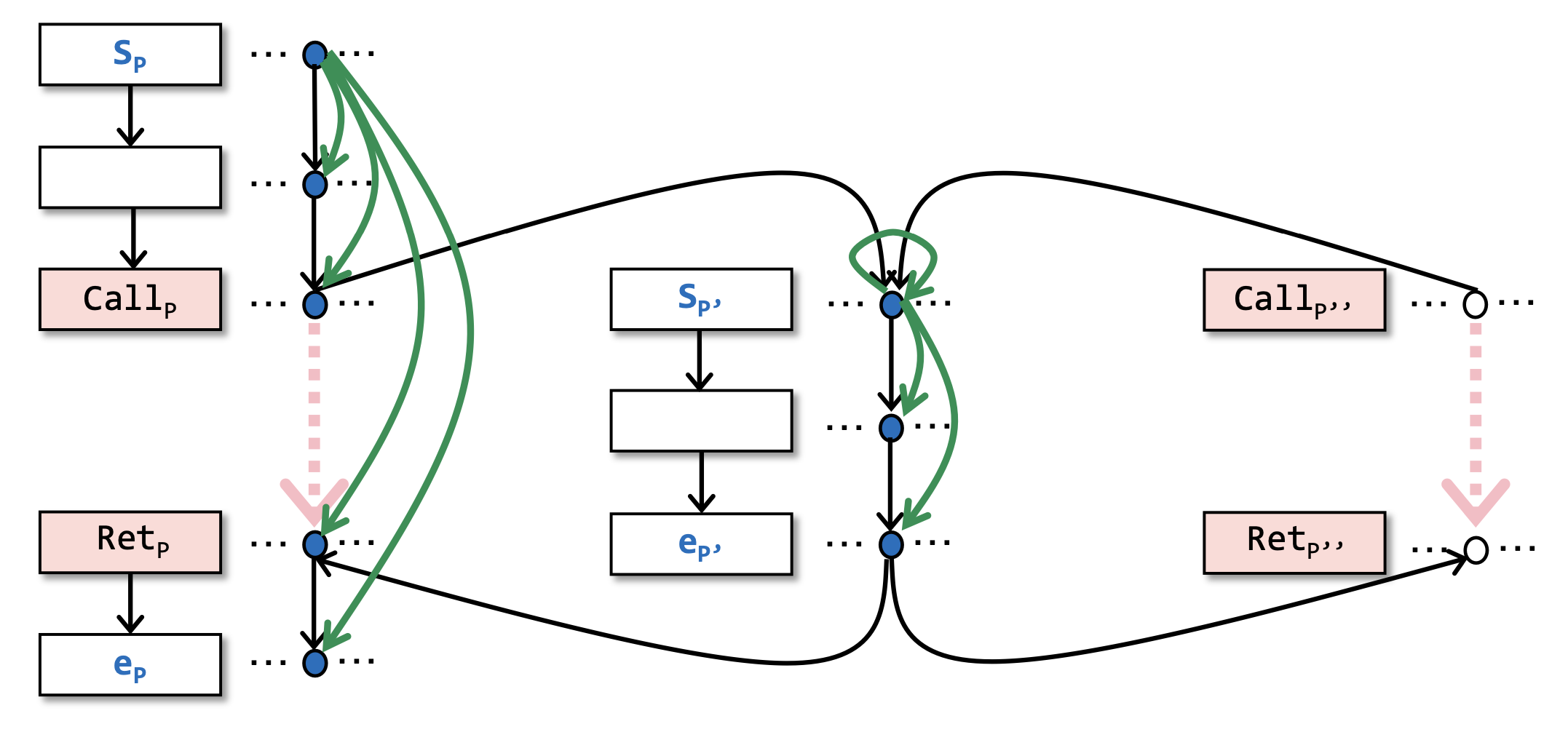1. 当图上的一个点 $(n, d)$ 从空心变成实心的时候，说明存在一条从 $(S_{main}, 0)$$(n, d)$ 的可实现路径（realizable path）。

## # 11.6 理解 IFDS 的分配性

### # 11.6.1 分配性的理解和判断

$F(x \wedge y) = F(x) \wedge F(y)$

### # 11.6.2 指针分析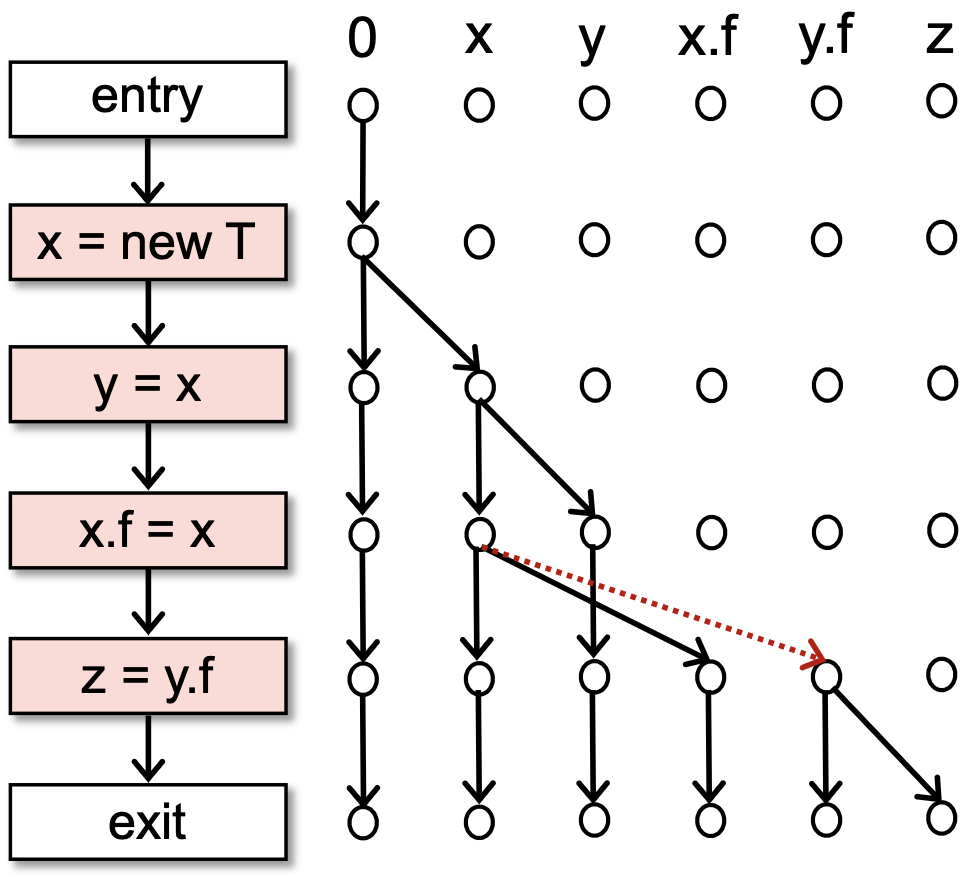## # 11.7 自检问题

1. 什么是CFL可达（CFL-Reachability）？

2. IFDS（Interprocedural Finite Distributive Subset Problem）的基本想法是什么？

3. 怎样的问题可以用IFDS来解决？